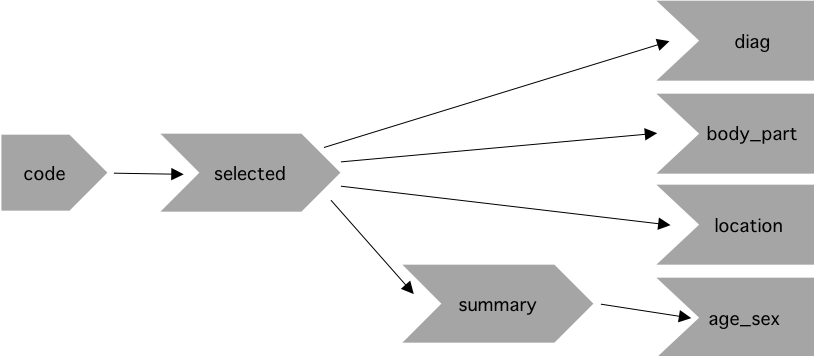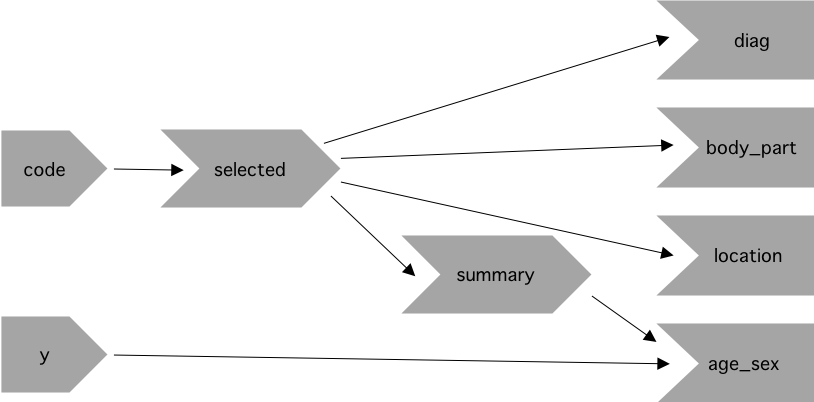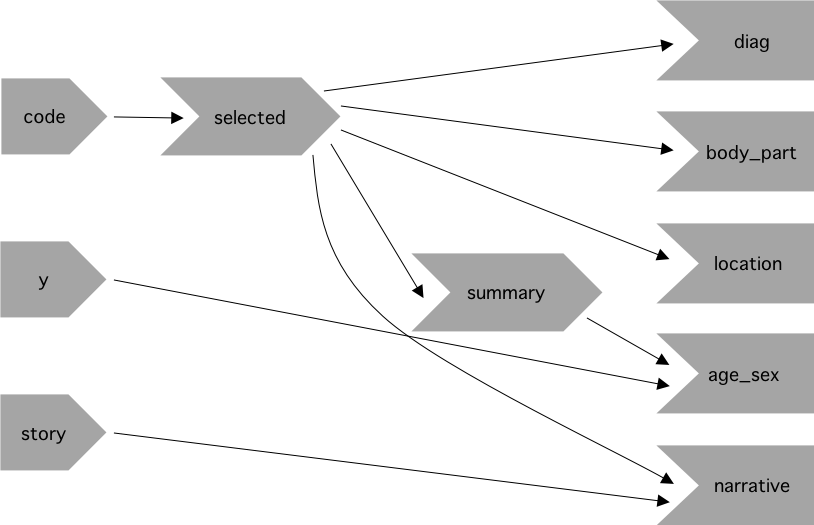# Chapter 5 Case Study: ER Injuries

### Exercise 5.8.1

Draw the reactive graph for each app.

#### Solution. Solution

##### Prototype

The prototype application has a single input, `input\$code`, which is used to generate the `selected()` reactive. This reactive is used directly in 3 outputs, `output\$diag`, `output\$body_part`, and `output\$location`, and it is also used indirectly in the `output\$age_sex` plot via the `summary()` reactive.##### Rate vs. Count

Building on the prototype, we create a second input `input\$y` which is used along with the `summary()` reactive to create the `output\$age_sex` plot.##### Narrative

Building on the application once more, we create an `output\$narrative` that depends on the `selected()` reactive and a new input, `input\$story`.### Exercise 5.8.2

What happens if you flip `fct_infreq()` and `fct_lump()` in the code that reduces the summary tables?

#### Solution. Solution

As in the book, we will use the datasets `injuries`, `products`, and `population` appearing here: https://github.com/hadley/mastering-shiny/blob/master/neiss/data.R.

Flipping the order of `fct_infreq()` and `fct_lump()` will only change the factor levels order. In particular, the function `fct_infreq()` orders the factor levels by frequency, and the function `fct_lump()` also orders the factor levels by frequency but it will only keep the top `n` factors and label the rest as `Other`.

Let us look at the top five levels in terms of count within the `diag` column in the `injuries` dataset:

``````injuries %>%
group_by(diag) %>%
count() %>%
arrange(-n) %>%
``````## # A tibble: 5 x 2
## # Groups:   diag 
##   diag                      n
##   <chr>                 <int>
## 1 Other Or Not Stated   44937
## 2 Fracture              43093
## 3 Laceration            39230
## 4 Strain, Sprain        37002
## 5 Contusion Or Abrasion 35259``````

If we apply `fct_infreq()` first, then it will reorder the factor levels in descending order as seen in the previous output. If afterwards we apply `fct_lump()`, then it will lump together everything after the nth most commonly seen level.

``````diag <- injuries %>%
mutate(diag = fct_lump(fct_infreq(diag), n = 5)) %>%
pull(diag)

levels(diag)``````
``````##  "Other Or Not Stated"   "Fracture"              "Laceration"
##  "Strain, Sprain"        "Contusion Or Abrasion" "Other"``````

Conversely, if we apply `fct_lump()` first, then it will label the most frequently seen factor level as “Other”. If afterwards we apply `fct_infreq()`, then it will label the first level as “Other” and not as “Other Or Not Stated”, which was the case for the previous code.

``````diag <- injuries %>%
mutate(diag = fct_infreq(fct_lump(diag, n = 5))) %>%
pull(diag)

levels(diag)``````
``````##  "Other"                 "Other Or Not Stated"   "Fracture"
##  "Laceration"            "Strain, Sprain"        "Contusion Or Abrasion"``````

### Exercise 5.8.3

Add an input control that lets the user decide how many rows to show in the summary tables.

#### Solution. Solution

Our function `count_top` is responsible for grouping our variables into a set number of factors, lumping the rest of the values into “Other”. The function has an argument `n` which is set to `5`. By creating a `numericInput` called `rows` we can let the user set the number of `fct_infreq` dynamically. However, because `fct_infreq` is the number of factors + `Other`, we need to subtract 1 from what the user selects in order to display the number of rows they input.

``````library(shiny)
library(forcats)
library(dplyr)
library(ggplot2)

# Note: these exercises use the datasets `injuries`, `products`, and
# `population` as created here:

count_top <- function(df, var, n = 5) {
df %>%
mutate({{ var }} := fct_lump(fct_infreq({{ var }}), n = n)) %>%
group_by({{ var }}) %>%
summarise(n = as.integer(sum(weight)))
}

ui <- fluidPage(
fluidRow(
column(8, selectInput("code", "Product",
choices = setNames(products\$prod_code, products\$title),
width = "100%")
),
column(2, numericInput("rows", "Number of Rows",
min = 1, max = 10, value = 5)),
column(2, selectInput("y", "Y Axis", c("rate", "count")))
),
fluidRow(
column(4, tableOutput("diag")),
column(4, tableOutput("body_part")),
column(4, tableOutput("location"))
),
fluidRow(
column(12, plotOutput("age_sex"))
),
fluidRow(
column(2, actionButton("story", "Tell me a story")),
column(10, textOutput("narrative"))
)
)

server <- function(input, output, session) {
selected <- reactive(injuries %>% filter(prod_code == input\$code))

# Find the maximum possible of rows.
max_no_rows <- reactive(
max(length(unique(selected()\$diag)),
length(unique(selected()\$body_part)),
length(unique(selected()\$location)))
)

# Update the maximum value for the numericInput based on max_no_rows().
observeEvent(input\$code, {
updateNumericInput(session, "rows", max = max_no_rows())
})

table_rows <- reactive(input\$rows - 1)

output\$diag <- renderTable(
count_top(selected(), diag, n = table_rows()), width = "100%")

output\$body_part <- renderTable(
count_top(selected(), body_part, n = table_rows()), width = "100%")

output\$location <- renderTable(
count_top(selected(), location, n = table_rows()), width = "100%")

summary <- reactive({
selected() %>%
count(age, sex, wt = weight) %>%
left_join(population, by = c("age", "sex")) %>%
mutate(rate = n / population * 1e4)
})

output\$age_sex <- renderPlot({
if (input\$y == "count") {
summary() %>%
ggplot(aes(age, n, colour = sex)) +
geom_line() +
labs(y = "Estimated number of injuries") +
theme_grey(15)
} else {
summary() %>%
ggplot(aes(age, rate, colour = sex)) +
geom_line(na.rm = TRUE) +
labs(y = "Injuries per 10,000 people") +
theme_grey(15)
}
})

output\$narrative <- renderText({
input\$story
selected() %>% pull(narrative) %>% sample(1)
})
}

shinyApp(ui, server)``````

### Exercise 5.8.4

Provide a way to step through every narrative systematically with forward and backward buttons.

Advanced: Make the list of narratives “circular” so that advancing forward from the last narrative takes you to the first.

#### Solution. Solution

We can add two buttons `prev_story` and `next_story` to iterate through the narrative. In addition, we can include a reactive value, `story`, that keeps track of the current position in the narrative. When the button `prev_story` is pressed, `story` decreases by one. Similarly, when the button `next_story` is pressed, `story` increases by one. To do the advanced part, we use the mod function. This allows us to keep `story` between 1 and the current narrative’s length, and simulate the “circular” motion.

``````library(shiny)
library(forcats)
library(dplyr)
library(ggplot2)

# Note: these exercises use the datasets `injuries`, `products`, and
# `population` as created here:

count_top <- function(df, var, n = 5) {
df %>%
mutate({{ var }} := fct_lump(fct_infreq({{ var }}), n = n)) %>%
group_by({{ var }}) %>%
summarise(n = as.integer(sum(weight)))
}

ui <- fluidPage(
fluidRow(
column(8, selectInput("code", "Product",
choices = setNames(products\$prod_code, products\$title),
width = "100%")
),
column(2, numericInput("rows", "Number of Rows",
min = 1, max = 10, value = 5)),
column(2, selectInput("y", "Y Axis", c("rate", "count")))
),
fluidRow(
column(4, tableOutput("diag")),
column(4, tableOutput("body_part")),
column(4, tableOutput("location"))
),
fluidRow(
column(12, plotOutput("age_sex"))
),
fluidRow(
column(2, actionButton("prev_story", "Previous story")),
column(2, actionButton("next_story", "Next story")),
column(8, textOutput("narrative"))
)
)

server <- function(input, output, session) {
selected <- reactive(injuries %>% filter(prod_code == input\$code))

# Find the maximum possible of rows.
max_no_rows <- reactive(
max(length(unique(selected()\$diag)),
length(unique(selected()\$body_part)),
length(unique(selected()\$location)))
)

# Update the maximum value for the numericInput based on max_no_rows().
observeEvent(input\$code, {
updateNumericInput(session, "rows", max = max_no_rows())
})

table_rows <- reactive(input\$rows - 1)

output\$diag <- renderTable(
count_top(selected(), diag, n = table_rows()), width = "100%")

output\$body_part <- renderTable(
count_top(selected(), body_part, n = table_rows()), width = "100%")

output\$location <- renderTable(
count_top(selected(), location, n = table_rows()), width = "100%")

summary <- reactive({
selected() %>%
count(age, sex, wt = weight) %>%
left_join(population, by = c("age", "sex")) %>%
mutate(rate = n / population * 1e4)
})

output\$age_sex <- renderPlot({
if (input\$y == "count") {
summary() %>%
ggplot(aes(age, n, colour = sex)) +
geom_line() +
labs(y = "Estimated number of injuries") +
theme_grey(15)
} else {
summary() %>%
ggplot(aes(age, rate, colour = sex)) +
geom_line(na.rm = TRUE) +
labs(y = "Injuries per 10,000 people") +
theme_grey(15)
}
})

# Store the maximum posible number of stories.
max_no_stories <- reactive(length(selected()\$narrative))

# Reactive used to save the current position in the narrative list.
story <- reactiveVal(1)

# Reset the story counter if the user changes the product code.
observeEvent(input\$code, {
story(1)
})

# When the user clicks "Next story", increase the current position in the
# narrative but never go beyond the interval [1, length of the narrative].
# Note that the mod function (%%) is keeping `current`` within this interval.
observeEvent(input\$next_story, {
story((story() %% max_no_stories()) + 1)
})

# When the user clicks "Previous story" decrease the current position in the
# narrative. Note that we also take advantage of the mod function.
observeEvent(input\$prev_story, {
story(((story() - 2) %% max_no_stories()) + 1)
})

output\$narrative <- renderText({
selected()\$narrative[story()]
})
}

shinyApp(ui, server)``````